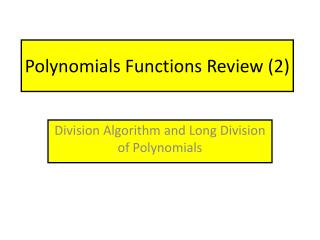Download PresentationPolynomials Functions Review (2)

# Polynomials Functions Review (2) - PowerPoint PPT Presentation

Polynomials Functions Review (2). Division Algorithm and Long Division of Polynomials. Example Set No.1. Find the quotient and remainder for each division problem. Be sure that the dividend and the divisor are written in descending order.I am the owner, or an agent authorized to act on behalf of the owner, of the copyrighted work described.
Download Presentation## Polynomials Functions Review (2)

Download Policy: Content on the Website is provided to you AS IS for your information and personal use and may not be sold / licensed / shared on other websites without getting consent from its author.While downloading, if for some reason you are not able to download a presentation, the publisher may have deleted the file from their server.

- - - - - - - - - - - - - - - - - - - - - - - - - - E N D - - - - - - - - - - - - - - - - - - - - - - - - - -
Presentation Transcript
1. Polynomials Functions Review (2) Division Algorithm and Long Division of Polynomials

2. Example Set No.1 Find the quotient and remainder for each division problem. Be sure that the dividend and the divisor are written in descending order. Account for each term in the dividend – physically write any missing term with a coefficient of 0. Rewrite the division problem in “long division” form

3. For the first step, divide the leading term of the divisor into the leading term of the dividend. The result is the first term of the quotient. Multiply this result by the divisor and subtract from the dividend. Remember that you are subtracting the entire product from the dividend.

4. Now divide the leading term of the divisor into the leading term of the current remainder. This is the next term of the quotient. Multiply this result by the divisor and subtract from the current remainder in the dividend.

5. Since the degree of the divisor is greater than the degree of the current remainder, the division is complete.

6. You can also write the result of the division in the form: Check your work

8. Noticing that the dividend is a difference of cubes, we know that we should obtain:

9. Since the remainder of the division is 0, we can conclude that is a factor of

10. Take a few minutes to complete this division before the solution is shown.

11. Remember to check your answers either by hand or by using the graphing calculator.

12. www.calc101.com

13. Synthetic Substitution and Synthetic Division

14. Direct Substitution Coefficients of polynomial function in descending order, filling in 0 for any “missing” term. Synthetic Substitution Value of x being substituted into the function.

15. First term drops straight down.

16. Direct Substitution Synthetic Substitution

17. Direct Substitution Synthetic Substitution

18. Why Synthetic Substitution Works Consider evaluating the following: Direct Substitution Synthetic Substitution

19. Synthetic Division The Factor Theorem The Remainder Theorem

20. The Factor Theorem The Factor Theorem is a formal statement of the Fundamental Connections that we made between zeros of a polynomial function and factors of the polynomial.

21. The Remainder Theorem When a polynomial is divided by x – a, then the remainder must either be 0 or a constant. Consider the division algorithm for dividing the polynomial function f (x) by x – a. Evaluating f (a), we have

22. The Remainder Theorem tells us that synthetic substitution can be used to not only evaluate a function, but also to perform polynomial division when a polynomial is divided by a divisor in the form x – a . When we interpret the process of synthetic substitution as the result of polynomial division, we typically refer to the process as synthetic division. The divisor in synthetic division MUST be of the form x – a. If a polynomial is divided by x + a, you must be thinking of the divisor as x – (–a).

23. Check the result with your calculator. Coefficients of the quotient, which is one degree less than the dividend. These are listed in descending order. Remainder.

24. Check the result with your calculator. Coefficients of the quotient, which is one degree less than the dividend. These are listed in descending order. Remainder.

25. Solution In the previous Example set, we found two zeros of this polynomial function, x = 2 and x = ‒1. Since we need to write a complete factorization of this polynomial function, we will examine a procedure that utilizes repeated steps of synthetic division to find this factorization.

26. From Example Set No.2, we have the following synthetic division: Now perform synthetic division again, using ‒1 on the quotient from above.

27. We now know that the polynomial will factor into the product of two linear factors and a quadratic factor.

28. Verify the zeros with your calculator. Viewing window: [‒5,3] by [‒8,6]